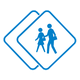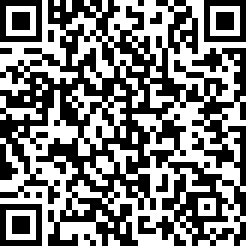#### ACT American College Testing (Exam 5)

created by Fisher BRink (@fisher) at Feb. 8, 2016
• In the diagram above, triangle ABC is a right triangle and the diameter of circle ...

• In a restaurant, the ratio of four-person booths to two-person booths is 3:5. If 1...

• If , what is the value of y when ?

• A pair of Jump sneakers costs \$60 and a pair of Speed sneakers costs \$45. For the ...

• In the diagram above, line AB is parallel to line CD, angle EIJ measures 140 degre...

• The area of one square face of a rectangular prism is 121 square units. If the vol...

• It takes six people eight hours to stuff 10,000 envelopes. How many people would b...

• In the diagram above of , for how many values does ?

• The equation

• In the diagram above, if angle OBE measures 110 degrees, what is the measure of ar...

• The volume of a cylinder is 486 cubic units. If the height of the cylinder is six ...

• Gil drives five times farther in 40 minutes than Warrick drives in 30 minutes. If ...

• In the diagram above, what is the area of the rectangle?

• If set M contains only the positive factors of 8 and set N contains only the posit...

• In the diagram above, ABCD is a square with an area of 100 c and lines BD and AC a...

• It takes eight people 12 hours to clean an office. How long would it take six peop...

• Greg has nine paintings. The Hickory Museum has enough space to display three of t...

• If the surface area of a cube is 384 cm2, what is the volume of the cube?

• In the diagram above, what is the sum of the measures of the angles x, y, and z?

• Given the following figure with one tangent and one secant drawn to the circle, wh...

Be the first to review

• info
Quiz Info
• date_range
Feb. 8, 2016, 3:34 a.m.
help_outline
20 questions
dvr
1 completed
remove_red_eye
15 views
people
0 takers
folder•#### Ratings

star_borderstar_borderstar_borderstar_borderstar_border
ratings